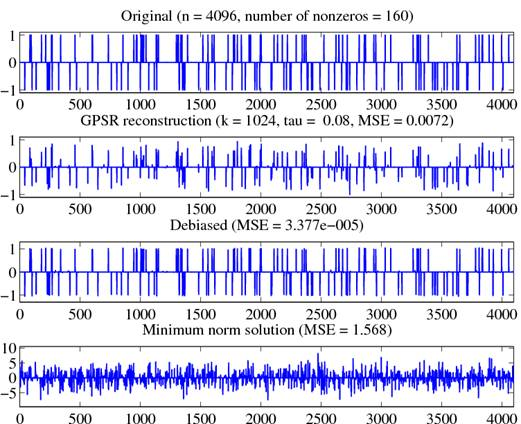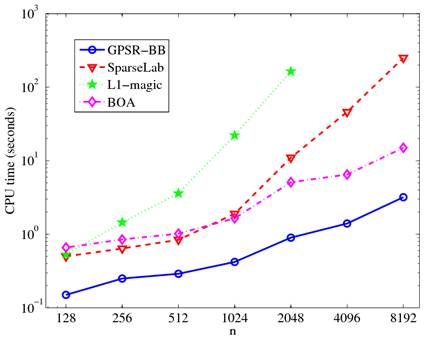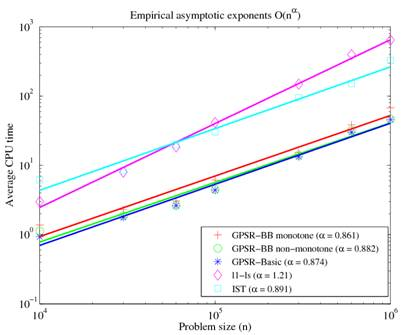GPSR

Instituto de Telecomunicações           Electrical and Computer Engineering                   Computer Sciences Dept.

Many problems in signal processing and statistical inference are based on finding a sparse solution to an undetermined linear system of equations.

Basis Pursuit, the Least Absolute Shrinkage and Selection Operator (LASSO), wavelet-based deconvolution, and Compressed Sensing are just a few well-known examples.

Computationally, the problem can be formulated in different ways, most of them being convex optimization problems. We considered a formulation in which a penalty term involving the scaled l1-norm of the signal is added to a least-squares term, a problem that can be reformulated as a convex quadratic program with bound constraints. This problem has a potentially extremely large number of variables (though only a small fraction of them are away from their bounds at the solution) and the data that defines it can often not be stored explicitly. We found that a solver of gradient projection type, using special line search and termination techniques, gave faster solutions on our test problems than other techniques that had been proposed previously, including interior-point techniques.  A debiasing step based on the conjugate-gradient algorithm improves the results further.

Final version,  September 12, 2007.

To appear in the IEEE Journal of Selected Topics in Signal Processing: Special Issue on Convex Optimization Methods for Signal Processing).

NEW!  Updated version (January 19, 2009) of the MATLAB code is available here: GPSR_6.0

The figure below shows a test case of a signal with 4096 elements only 160 of which are not zero, and which is being reconstructed from projection on 1024 unit-norm random vectors in 4096-dimensional space; this is, of course, a highly under-determined problem.  The true signal is shown at the top, while the reconstructions obtained from the l1-regularized formulation are shown in the second and third plots. Note that the locations of the spikes are reconstructed with high accuracy; their magnitudes are attenuated, but these can be corrected by applying our conjugate-gradient debiasing approach. The lower part of the figure shows the minimum norm solution, which is not sparse and which bears little relation to the true signal.The figures below shows a comparison, in terms of computational speed, of GPSR versus three state-of-the-art solvers for the same problem:

·         the l1-magic code, available here (from CalTech);

·         the SparseLab code, available here (from Stanford);

·         the new l1_ls code (March 2007), available here (from Stanford);

·         the bound-optimization method (or iterative shrinkage/thresholding – IST), originally developed for wavelet-based deconvolution, described here.

The plots shows that our GPSR method is faster and scales more favorably

(w.r.t. n, the length of the unknown signal) than the competing techniques.

See the paper for details about the experiments.Funding Acknowledgment:

·         This work was partially supported by the USA National Science Foundation (NSF), under grants CCF-0430504  and  CNS-0540147.

·         This work was partially supported by the Portuguese Fundação para a Ciência e Tecnologia (FCT), under project POSC/EEA-CPS/61271/2004.